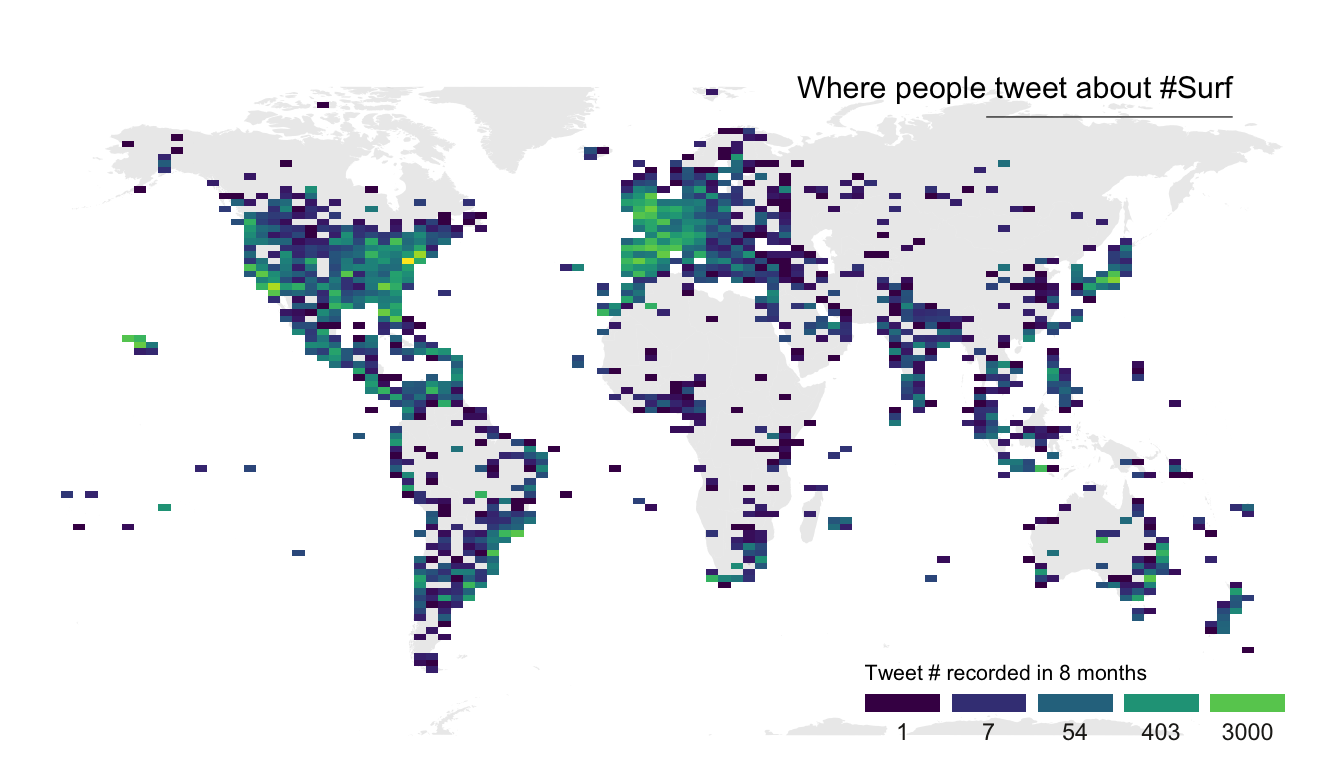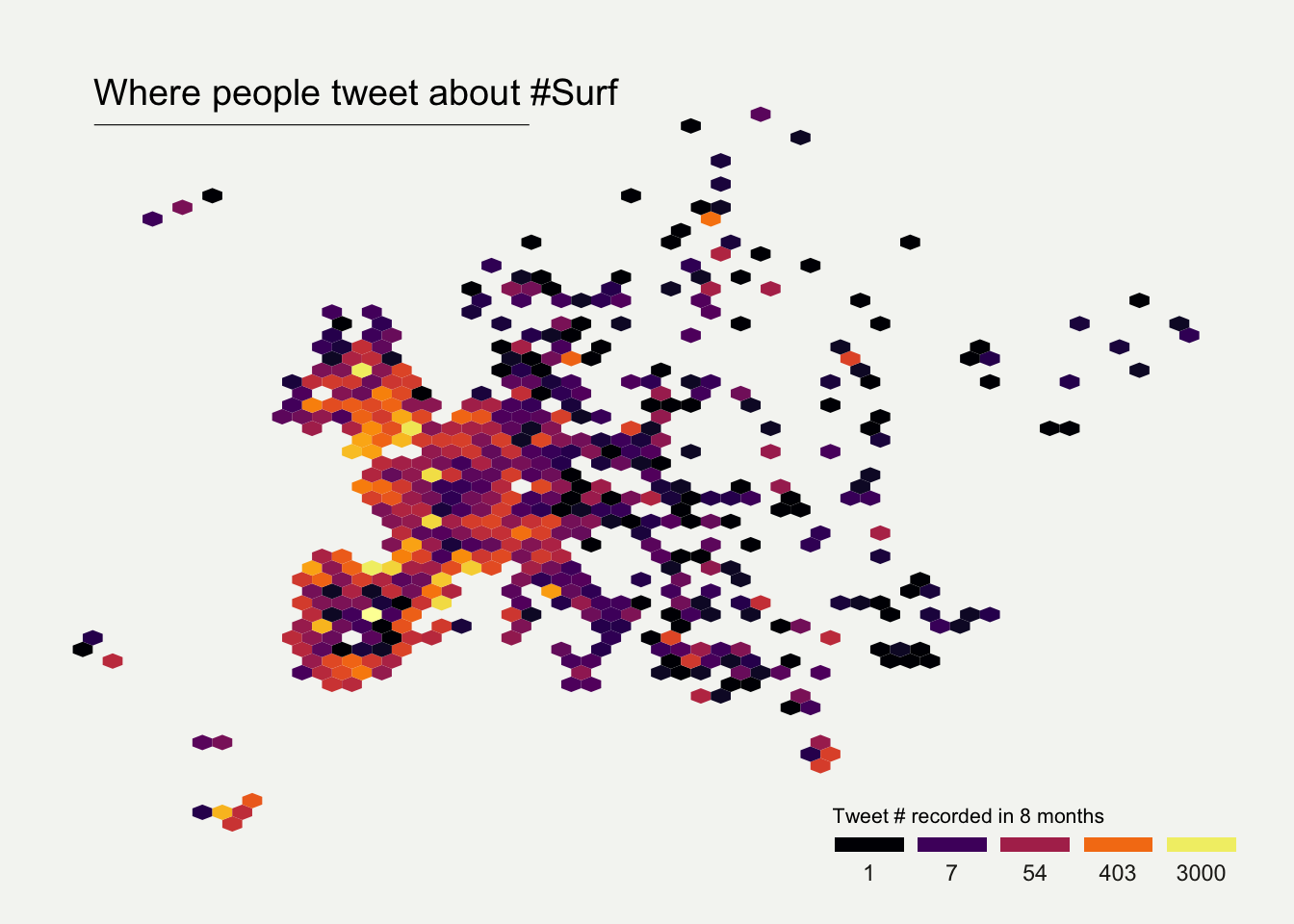# Hexbin map for 2D density visualization

It is possible to apply 2d density visualization methods on map to study the geographical distribution of a variable. The two most famous techniques beeing Hexbin maps and 2d histogram maps. This post describes how to build it with `R` and `ggplot2`.

Several techniques exist to visualize the distribution of two variables in the same time (2D distribution). The R graph gallery dedicates a whole section to it.

The idea is to count the number of observation within a particular area of the 2D space and represent this count by a color. This can method can be applied to maps using hexagones or squares, resulting in hexbin maps and 2d histogram maps.

In this post a list of GPS coordinates is used as input data. It comes from a project that harvested and geocoded a list of 200k tweets talking about #surf.

# 2d histogram maps

For 2d histogram maps the globe is split in several squares, the number of tweet per square is counted, and a color is attributed to each square.

• `ggplot2` offers the `geom_bin2d()` function that does all the calculation for us and plot the squares.
• the `geom_polygon()` function is used to show the world map in the background.
• `annotate()` is used to add a title.
• the `guide` option of `scale_fill_viridis` is used to create a nice legend.``````# Libraries
library(tidyverse)
library(viridis)
library(hrbrthemes)
library(mapdata)

# Get the world polygon
world <- map_data("world")

# plot
ggplot(data, aes(x=homelon, y=homelat)) +
geom_polygon(data = world, aes(x=long, y = lat, group = group), fill="grey", alpha=0.3) +
geom_bin2d(bins=100) +
ggplot2::annotate("text", x = 175, y = 80, label="Where people tweet about #Surf", colour = "black", size=4, alpha=1, hjust=1) +
ggplot2::annotate("segment", x = 100, xend = 175, y = 73, yend = 73, colour = "black", size=0.2, alpha=1) +
theme_void() +
ylim(-70, 80) +
scale_fill_viridis(
trans = "log",
breaks = c(1,7,54,403,3000),
name="Tweet # recorded in 8 months",
guide = guide_legend( keyheight = unit(2.5, units = "mm"), keywidth=unit(10, units = "mm"), label.position = "bottom", title.position = 'top', nrow=1)
)  +
ggtitle( "" ) +
theme(
legend.position = c(0.8, 0.09),
legend.title=element_text(color="black", size=8),
text = element_text(color = "#22211d"),
plot.title = element_text(size= 13, hjust=0.1, color = "#4e4d47", margin = margin(b = -0.1, t = 0.4, l = 2, unit = "cm")),
) ``````

# Hexbin maps

Hexbin maps are done using pretty much the same code.

Here, `geom_hex()` is used to create the hexagones. Note the `bins` option allowing to control the bin size, and thus the hexagone size on the map.``````# Libraries
library(tidyverse)
library(viridis)
library(hrbrthemes)
library(mapdata)

# plot
data %>%
filter(homecontinent=='Europe') %>%
ggplot( aes(x=homelon, y=homelat)) +
geom_hex(bins=59) +
ggplot2::annotate("text", x = -27, y = 72, label="Where people tweet about #Surf", colour = "black", size=5, alpha=1, hjust=0) +
ggplot2::annotate("segment", x = -27, xend = 10, y = 70, yend = 70, colour = "black", size=0.2, alpha=1) +
theme_void() +
xlim(-30, 70) +
ylim(24, 72) +
scale_fill_viridis(
option="B",
trans = "log",
breaks = c(1,7,54,403,3000),
name="Tweet # recorded in 8 months",
guide = guide_legend( keyheight = unit(2.5, units = "mm"), keywidth=unit(10, units = "mm"), label.position = "bottom", title.position = 'top', nrow=1)
)  +
ggtitle( "" ) +
theme(
legend.position = c(0.8, 0.09),
legend.title=element_text(color="black", size=8),
text = element_text(color = "#22211d"),
plot.background = element_rect(fill = "#f5f5f2", color = NA),
panel.background = element_rect(fill = "#f5f5f2", color = NA),
legend.background = element_rect(fill = "#f5f5f2", color = NA),
plot.title = element_text(size= 13, hjust=0.1, color = "#4e4d47", margin = margin(b = -0.1, t = 0.4, l = 2, unit = "cm")),
) ``````

Related chart types

## Contact

This document is a work by Yan Holtz. Any feedback is highly encouraged. You can fill an issue on Github, drop me a message on Twitter, or send an email pasting yan.holtz.data with gmail.com.